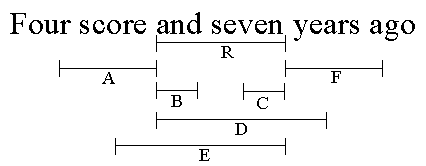Next: Relational Operators Up: Text Constraints Previous: Primitives

## Region Relations

TC operators are based on six fundamental binary relations among regions: before, after, in, contains, overlaps-start, and, overlaps-end. (Similar relations on time intervals were defined in .) The region relations are defined as follows:

 [b1,e1] before [b2,e2] if and only if e1 <= b2 [b1,e1] after [b2,e2] if and only if e2 <= b1 [b1,e1] in [b2,e2] if and only if b2 <= b1 and e1 <= e2 [b1,e1] contains [b2,e2] if and only if b1 <= b2 and e2 <= e1 [b1,e1] overlaps-start [b2,e2] if and only if b1 <= b2 and e1 <= e2 [b1,e1] overlaps-end [b2,e2] if and only if b2 <= b1 and e2 <= e1
Note that before and after are inverses, as are in and contains, and overlaps-start and overlaps-end. The six region relations are illustrated in Figure 2.Figure 2: Fundamental region relations in an example string. Regions A through F are related to region R as follows: A before R; B overlaps-start R; C contains R; D in R; E overlaps-end R; and F after R.
The six region relations are complete in the sense that every ordered pair of regions is found in at least one of the relations. Some regions may be related in several ways, however. For example, in Figure 2, if A's end point were identical to R's start point, then we would have both A before R and A overlaps-start R. These relations are useful in pattern matching, so we define a set of derived relations in which regions have coincident endpoints:
 just-before = before and overlaps-start just-after = after and overlaps-end at-start-of = in and overlaps-start at-end-of = in and overlaps-end starts-with = contains and overlaps-start ends-with = contains and overlaps-end
Figure 3 illustrates the derived relations.Figure 3: Region relations with coincident endpoints. Regions A through F are related to region R as follows: A just-before R; B at-start-of R; C at-end-of R; D starts-with R; E ends-with R; and F just-after R.
Another useful derived relation is overlaps:
 overlaps = in and contains and overlaps-start and overlaps-end
In Figure 2, the regions B, C, D, and E overlap R, but A and F do not. In Figure 3, all the regions overlap R.

Robert C. Miller and Brad A. Myers
Mon Apr 26 11:34:19 EDT 1999